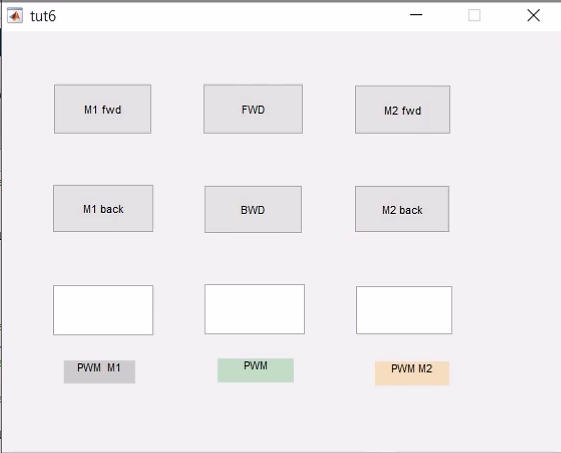# 1.5.0 PROJECT OVERVIEW: Interfacing L298N motor driver with Arduino using Matlab GUI

In this tutorial, we are going to interface L298N motor driver ic with arduino using this matlab GUI. So that we can control two motors either individually or together.

ALL MATLAB GUI TUTORIALS:

# 1.0 Installing Arduino support package & Matlab GUI basics in 2017

Welcome to the fifth tutorial or tutorial no. 1.5 of the course “using MATLAB GUI with Arduino support package”.Click here TUTORIAL 1.4  to read previous tutorial.

Watch video version of this project for better understanding:

Now since this tutorial is going to be a long one, we will learn this project step by step in separate
videos:

STEP1: Overview of this project
First STEP is to get the complete idea of the project which is this video, ofcourse.

STEP2: Creating required MATLAB GUI for project
In step two we will create this GUI i.e, GUI functions, visual appearance and all.

STEP3: Code for GUI
Then in step three we will actually write the code for this gui to work on Arduino so that L298N CAN be interfaced with arduino.

STEP4: Circuit Connections
In video 4 or Step 4 we will make the circuit connections of Arduino, L28N and motors.

STEP5: GUI in action!
And finally in STEP 5 we will see all above steps in action!

Now in this GUI there are three sections left, middle and rightmost. Left one is for controlling left motor , right one to control right motor and middle one to control both motor simultaneously. Top row of buttons is for clockwise direction and middleone is for anticlockwise rotation. And this last row in which all widgets are edit text boxes, we will type voltage value that we want across motor depending on which section we want to control.